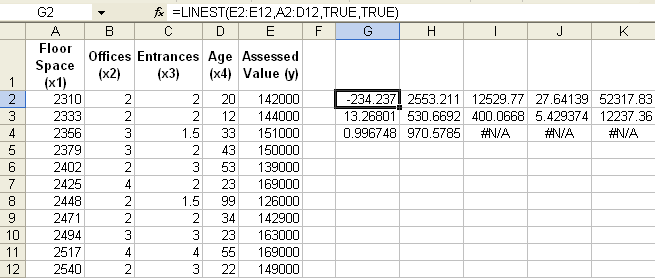# Using Formulas or Functions to Process Data

Contents
[ ]

## Using Formulas and Functions

Aspose.Cells provides a class, Workbook, that represents a Microsoft Excel file. The Workbook class contains a Worksheets collection that allows access to each worksheet in the Excel file. A worksheet is represented by the Worksheet class. The Worksheet class provides a Cells collection. Each item in the Cells collection represents an object of the Cell class.

It is possible to apply formulas to cells using properties and methods offered by the Cell class, discussed in more detail below.

## Using Built-in Functions

Built-in functions or formulas are provided as ready-made functions to reduce developers' efforts and time. See a list of built-in functions. The functions are listed in alphabetical order. More functions will be supported in the future.

Aspose.Cells supports most of the formulas or functions offered by Microsoft Excel. Developers can use these formulas through the API or designer spreadsheet. Aspose.Cells supports a huge set of mathematical, string, Boolean, date/time, statistical, database, lookup, and reference formulas.

Use the Formula property of the Cell class to add a formula to a cell. Complex formulas, for example

`` = H7*(1+IF(P7 = \$L\$3,\$M\$3, (IF(P7=\$L\$4,\$M\$4,0))))``

, are also supported in Aspose.Cells. When applying a formula to a cell, always begin the string with an equal sign (=) as you do when creating a formula in Microsoft Excel and use a comma (,) to delimit function parameters.

In the example below, a complex formula is applied to the first cell of a worksheet’s Cells collection. The formula uses a built-in IF function provided by Aspose.Cells.

We can have some user-defined formulas that we want to include as an excel add-in. When setting the Cell.Formula function built-in functions work fine however there is a need to set the custom functions or formulas using the add-in functions.

Aspose.Cells provides features to register add in functions using Worksheets.RegisterAddInFunction(). Afterwards when we set Cell.Formula = anyFunctionFromAddIn, the output Excel file contains the calculated value from the AddIn function.

Following the XLAM file shall be downloaded for registering the add-in function in the below sample code. Similarly, the output file “test_udf.xlsx” can be downloaded to check the output.

TestUDF.xlam

test_udf.xlsx

## Using Array Formula

Array formulas are formulas that work with arrays, instead of individual numbers, as arguments to the functions that make up the formula. When an array formula is displayed, it is surrounded by braces ({}) as shown below.

Setting an array formula on cell G2Some Microsoft Excel functions return arrays of values. To calculate multiple results with an array formula, enter the array into a range of cells with the same number of rows and columns as the array arguments.

It is possible to apply an array formula to a cell by calling the Cell class' setArrayFormula method. The setArrayFormula method takes the following parameters:

• Array Formula, the array formula.
• Number of Rows, the number of rows to populate result of the array formula.
• Number of Columns, the number of columns to populate the result of the array formula.

## Using R1C1 Formula

Apply an R1C1 reference style formula to a cell with the Cell class' setR1C1Formula property.